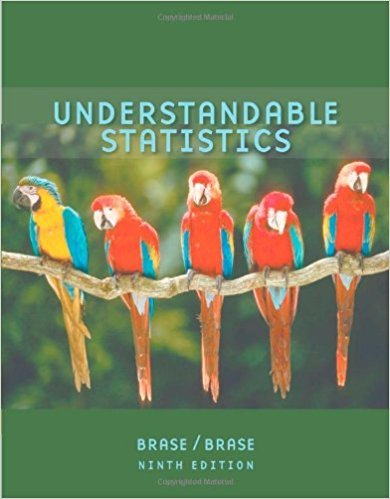×
×

# Solutions for Chapter 12.4: NONPARAMETRIC STATISTICS## Full solutions for Understandable Statistics | 9th Edition

ISBN: 9780618949922Solutions for Chapter 12.4: NONPARAMETRIC STATISTICS

Solutions for Chapter 12.4
4 5 0 366 Reviews
15
4
##### ISBN: 9780618949922

This expansive textbook survival guide covers the following chapters and their solutions. Since 12 problems in chapter 12.4: NONPARAMETRIC STATISTICS have been answered, more than 35900 students have viewed full step-by-step solutions from this chapter. Understandable Statistics was written by and is associated to the ISBN: 9780618949922. Chapter 12.4: NONPARAMETRIC STATISTICS includes 12 full step-by-step solutions. This textbook survival guide was created for the textbook: Understandable Statistics, edition: 9.

Key Statistics Terms and definitions covered in this textbook
• `-error (or `-risk)

In hypothesis testing, an error incurred by rejecting a null hypothesis when it is actually true (also called a type I error).

• Acceptance region

In hypothesis testing, a region in the sample space of the test statistic such that if the test statistic falls within it, the null hypothesis cannot be rejected. This terminology is used because rejection of H0 is always a strong conclusion and acceptance of H0 is generally a weak conclusion

• Bivariate distribution

The joint probability distribution of two random variables.

• Block

In experimental design, a group of experimental units or material that is relatively homogeneous. The purpose of dividing experimental units into blocks is to produce an experimental design wherein variability within blocks is smaller than variability between blocks. This allows the factors of interest to be compared in an environment that has less variability than in an unblocked experiment.

• Center line

A horizontal line on a control chart at the value that estimates the mean of the statistic plotted on the chart. See Control chart.

• Coeficient of determination

See R 2 .

• Conidence level

Another term for the conidence coeficient.

• Correlation

In the most general usage, a measure of the interdependence among data. The concept may include more than two variables. The term is most commonly used in a narrow sense to express the relationship between quantitative variables or ranks.

• Decision interval

A parameter in a tabular CUSUM algorithm that is determined from a trade-off between false alarms and the detection of assignable causes.

• Deining relation

A subset of effects in a fractional factorial design that deine the aliases in the design.

• Dependent variable

The response variable in regression or a designed experiment.

• Design matrix

A matrix that provides the tests that are to be conducted in an experiment.

• Designed experiment

An experiment in which the tests are planned in advance and the plans usually incorporate statistical models. See Experiment

• Discrete uniform random variable

A discrete random variable with a inite range and constant probability mass function.

• Empirical model

A model to relate a response to one or more regressors or factors that is developed from data obtained from the system.

• Error propagation

An analysis of how the variance of the random variable that represents that output of a system depends on the variances of the inputs. A formula exists when the output is a linear function of the inputs and the formula is simpliied if the inputs are assumed to be independent.

• Estimate (or point estimate)

The numerical value of a point estimator.

• Fisher’s least signiicant difference (LSD) method

A series of pair-wise hypothesis tests of treatment means in an experiment to determine which means differ.

• Forward selection

A method of variable selection in regression, where variables are inserted one at a time into the model until no other variables that contribute signiicantly to the model can be found.

• Geometric mean.

The geometric mean of a set of n positive data values is the nth root of the product of the data values; that is, g x i n i n = ( ) = / w 1 1 .

×